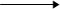Visitors Online: 77 | Saturday 23rd March 2019CBSE Guess > Papers > Important Questions > Class XII > 2013 > Chemistry > Electrochemistry by Mr. R. Srinivas Vasudevamurthy CBSE CLASS XII
Electrochemistry - 5 Marks Questions 1. Mention the differences between electrolytic cell and electrochemical cell. 2. Explain the terms a) electrode potential b) standard electrode potential c) cell 3. potential d) electromotive force of a cell. 4. what will happen when a) Eext = 1.10 V b) Eext < 1.10 V c) Eext > 1.10 V is applied on the cell Zn / Zn2+ || Cu2+/ Cu ? 5.What is SHE ? How will you determine the potential of a) Zn electrode b) Cu electrode using SHE ? 6. E0 Zn2+ / Zn = - 0.76 V and . E0 Cu2+ / Cu = + 0.34 V. What does positive and negative sign convey ? 7. Account for the following: a) Zn displaces hydrogen from dilute HCl while Cu can not. b) Li is the strongest reducing agent while Fluorine is strongest oxidising agent. c) copper sulphate solution can not be stored in zinc vessel. E0 Cu2+ / Cu = + 0.34 V E0 Zn2+ / Zn = - 0.76 VE0 Li+ / Li = - 3.05 V E0 F2 /2 F- = + 2.87 V) 8. Which of the following reactions are feasible? a) Fe3+(aq) and I- (aq) b) Fe3+(aq) and Br- (aq) (E0 Fe3+ / Fe2+ = 0.77 V, E0 I2 /2 I- = 0.54 V, E0 Br2 /2 Br- = 1.09 V) 9. Write Nernst equation for the following cells at 298 K a) Cr/ Cr3+     ||   Fe2+ / Fe       ( 0.1M)           ( 0.1M) b) Zn + Sn4+Zn2+  + Sn2+      (1.5M)                             (0.5M)(2M) 10. Explain the terms a) resistance b) resistivity c) conductance d) conductivity e) molar conductivity. 11. What is the effect of temperature on a) electrolytic conductivity b) metallic conductivity? 12. How does conductivity of an electrolyte solution varies with the dilution? 13. How does molar conductivity of an electrolyte solution varies with the dilution ? 14. Write the Kohlrausch equation and draw a graph to show the variation of molar conductivity with Öc for a) KCl b) CH3COOH. 15. State Kohlrausch law of independent migration of ions.. Mention two applications of the law. 16. Give the products of electrolysis of a) NaCl (molten) b) NaCl (aq) c) H2SO4(aq) d) CuSO4(aq) using inert electrode like Pt e) CuSO4(aq) using Cu electrodes f) AgNO3(aq) using Ag electrode g) AgNO3(aq) using Pt electrode. 17. Write the reactions involved at each electrode in Leclanche cell. Mention the disadvantage of this cell. 18. Write the reactions involved at each electrode in mercury cell. Why does the cell potential of this cell remains constant? 19. What are fuel cells? Write the reactions involved at each electrode in H2-O2 fuel cell. 20. Give one similarity and one difference between fuel cell and other primary cells. 21. What are secondary cells? Write the reactions involved at each electrode in lead storage cell when a) battery is in use b) battery is not in use. 22. Write the equation of the overall reaction in Ni-Cd cell. during discharge. 23. Explain corrosion of iron as a electrochemical process. Mention the methods to prevent corrosion. 24. Iron bar A is coated with Zn and another bar B is coated with Sn. Which will rust faster when the coating is broken ?. 25. State Faraday’s laws of electrolysis. What is Faraday constant?   Submitted By : Mr. R. Srinivas Vasudevamurthy Email: vasudm@yahoo.co.uk